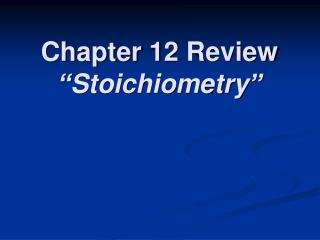DownloadDownload PresentationChapter 12 Review “Stoichiometry”

# Chapter 12 Review “Stoichiometry”

Télécharger la présentation## Chapter 12 Review “Stoichiometry”

- - - - - - - - - - - - - - - - - - - - - - - - - - - E N D - - - - - - - - - - - - - - - - - - - - - - - - - - -
##### Presentation Transcript

1. Chapter 12 Review“Stoichiometry”

2. Chapter 12 Review • The first step in most stoichiometry problems is to ____. • How many liters of hydrogen gas are needed to react with CS2 to produce 2.50 L of CH4 at STP: 4H2(g) + CS2(l)→ CH4(g) + 2H2S(g)? • In a chemical reaction, the mass of the products ____.

3. Chapter 12 Review • In the following reaction: 2CO(g) + O2(g)→ 2CO2(g), what is the ratio of moles of oxygen used to moles of CO2 produced? • When iron rusts in air, iron (III) oxide is produced. How many moles of oxygen react with 2.4 mol of iron in the rusting reaction: 4Fe(s) + 3O2(g) → 2Fe2O3(s)

4. Chapter 12 Review • Which of the following is true for: C5H12(l) + 8O2(g)→ 5CO2(g) + 6H2O(l) a) 9 moles of reactants chemically change into 11 moles of product, or b) 9 atoms of reactants chemically change into 11 atoms of product? • How many moles of aluminum react with 1.2 mol of FeO: 2Al(s) + 3FeO(s) → 3Fe(s) + Al2O3(s)?

5. Chapter 12 Review • Which of the following is NOT true about limiting and excess reagents: a) the reactant that has the smallest given mass is the limiting reagent, or b) some of the excess reagent is left over after the reaction is complete? • In every chemical reaction, ____ are conserved.

6. Chapter 12 Review • Which of the following is an incorrect interpretation of the equation 2S(s) + 3O2(g)→ 2SO3(g): a) 2 atoms S + 3 molecules O2 → 2 molecules SO3, or b) 2 g S + 3 g O2 → 2 g SO3? • The calculation of quantities in chemical equations is called ____. • What is conserved in this reaction: N2(g) + 3F2(g) → 2NF3(g)?

7. Chapter 12 Review • When 0.1 mol of Ca reacts with 880 g water, 2.24 L of hydrogen gas forms (at STP). How would the amount of hydrogen produced change if the volume of water was decreased to 440 mL (440 g)? • When two substances react to form products, the reactant which is used up is called the ____.

8. Chapter 12 Review • Which of the following is NOT true about “yield”: a) the value of actual yield must be given to calculate percent yield, or b) the actual yield may be different from the theoretical yield because insufficient limiting reagent was used?

9. Chapter 12 Review • If 1 egg and 1/3 cup of oil are needed for each bag of brownie mix, how many bags of brownie mix do you need if you want to use up 3 eggs and 1 cup of oil? • In a reaction between copper metal and silver nitrate, 12.7 g Cu produced 38.1 g Ag. What is the percent yield of silver in this reaction?

10. Chapter 12 Review • Which of the following is true about 3NaHCO3 + C6H8O7→ 3CO2 + 3H2O + Na3C6H5O7: a) 22.4 L CO2 are produced for every liter of C6H8O7 reacted, or b) 1 mole of water is produced for every mole of carbon dioxide produced? • Which type of stoichiometric calculation does not require the use of the molar mass?

11. Chapter 12 Review • Which of the following is NOT a reason why actual yield is less than theoretical yield: a) impure reactant present, or b) conservation of mass? • What is conserved in this reaction: H2(g) + Cl2(g)→ 2HCl(g)? • In any chemical reaction, the quantities that are preserved are __.

12. Chapter 12 Review • When an equation is used to calculate the amount of product that will form during a reaction, then the value obtained is called the ____. • How many grams of NaCl could be produced from 103.0 g Na reacting with 13.0 L of Cl2 at STP?

13. Chapter 12 Review • How many grams of CO are needed to react with an excess of Fe2O3 to produce 209.7 g Fe? Fe2O3(s) + 3CO(g)→ 3CO2(g) + 2Fe(s) • How many liters of O2 are needed to react completely with 45.0 L of H2S at STP? 2H2S(g) + 3O2(g) → 2SO2(g) + 2H2O(g)

14. Chapter 12 Review • If 8.6 L of H2 reacted with 4.3 L of O2 at STP, what is the volume of the gaseous water collected? 2H2(g) + O2(g)→ 2H2O(g) • If 8.00 mol of NH3 reacted with 14.0 mol of O2, how many moles of H2O will be produced? 4NH3(g) + 7O2(g) → 4NO2(g) + 6H2O(g)

15. Chapter 12 Review • If a tricycle factory ordered 33,432 wheels in 2002 and used all of them, how many tricycles did the factory produce? • If O2 was the excess reagent, 8.3 mol of H2S were consumed, and 137.1 g of water were collected, what is the percent yield of this reaction? 2H2S(g) + 3O2(g) → 2SO2(g) + 2H2O(g)

16. Chapter 12 Review End of Chapter 12 Review